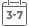# A First Course in Mathematical Logic and Set Theory

• Publish Date: 2015-09-08
• Binding: Hardcover
• Author: Michael L. O'Leary
• Sale
• Regular price \$161.65A mathematical introduction to the theory and applications of logic and set theory with an emphasis on writing proofs

Highlighting the applications and notations of basic mathematical concepts within the framework of logic and set theory, A First Course in Mathematical Logic and Set Theory introduces how logic is used to prepare and structure proofs and solve more complex problems.

The book begins with propositional logic, including two-column proofs and truth table applications, followed by first-order logic, which provides the structure for writing mathematical proofs. Set theory is then introduced and serves as the basis for defining relations, functions, numbers, mathematical induction, ordinals, and cardinals. The book concludes with a primer on basic model theory with applications to abstract algebra. A First Course in Mathematical Logic and Set Theory also includes:

• Section exercises designed to show the interactions between topics and reinforce the presented ideas and concepts
• Numerous examples that illustrate theorems and employ basic concepts such as Euclids lemma, the Fibonacci sequence, and unique factorization
• Coverage of important theorems including the well-ordering theorem, completeness theorem, compactness theorem, as well as the theorems of LwenheimSkolem, Burali-Forti, Hartogs, CantorSchrderBernstein, and Knig

An excellent textbook for students studying the foundations of mathematics and mathematical proofs, A First Course in Mathematical Logic and Set Theory is also appropriate for readers preparing for careers in mathematics education or computer science. In addition, the book is ideal for introductory courses on mathematical logic and/or set theory and appropriate for upper-undergraduate transition courses with rigorous mathematical reasoning involving algebra, number theory, or analysis.

MORE FROM THIS COLLECTION